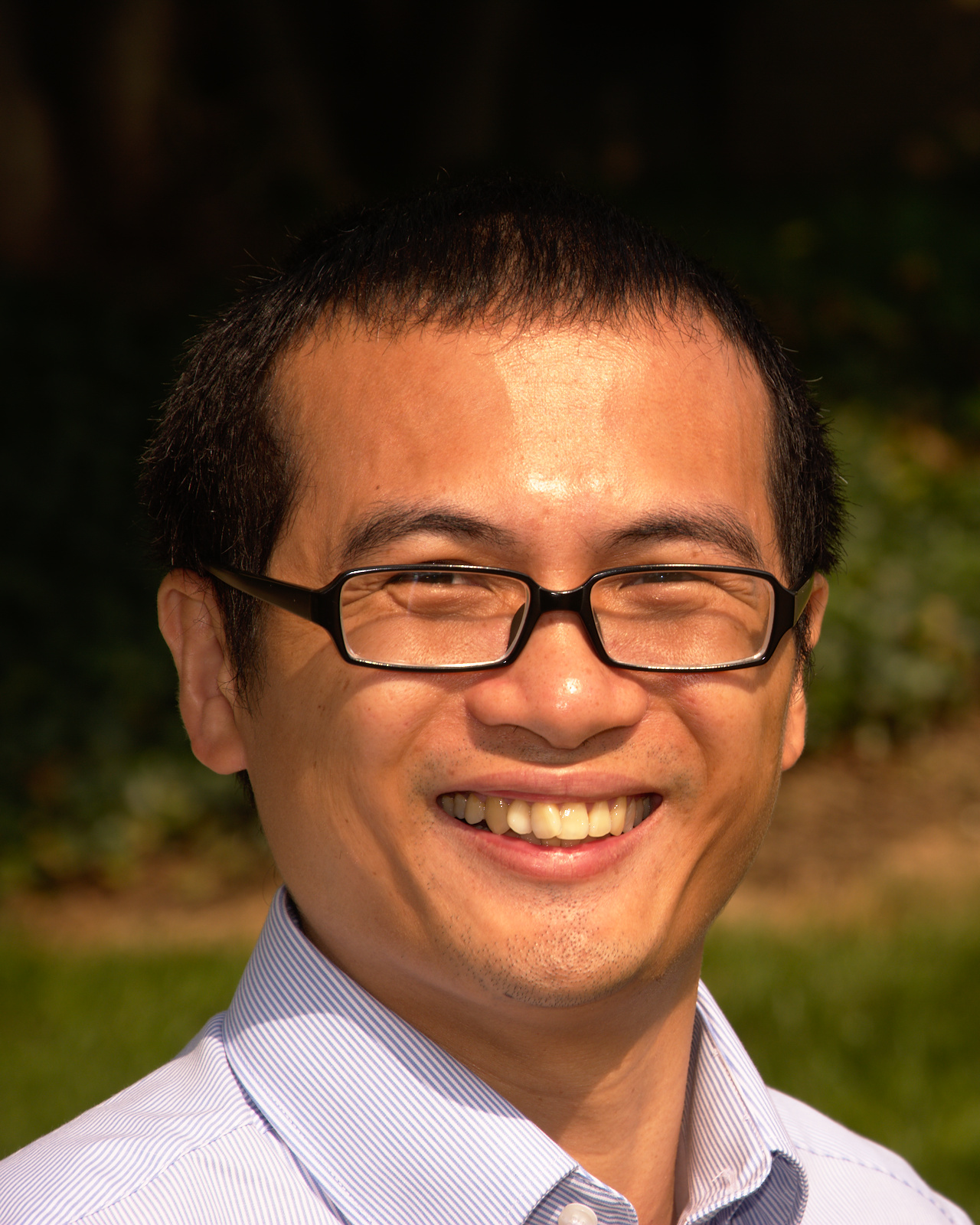# Welcome to Trieu Le's homepage(picture taken by Nate Iverson)
Dept. of Mathematics and Statistics
University of Toledo
Toledo, OH 43606
• Curriculum Vitae (pdf)
• My full name in Vietnamese is Lê Long Triều. I received my Ph.D. in Mathematics from the University at Buffalo in 2007 under the direction of Prof. Jingbo Xia. I spent the year 2007-2008 as a postdoctoral fellow at the University of Toronto and the Fields Institute under the supervision of Prof. George Elliott. During 2008-2009, I was a postdoctoral fellow at the University of Waterloo under the supervision of Prof. Ken Davidson. I've been at the University of Toledo since 2009.
• Email: trieu (dot) le (number 2) (at) utoledo (dot) edu
• Teaching Statement (pdf)
• I've taught a variety of courses from introductory undergraduate to graduate courses. Below is a list of courses that I have taught at UT and elsewhere.
• For students: take a Calculus 1 Quiz.

• MATH 4830/5830 (Intro to Real Analysis II) and MATH 6980/8980 (Topics in Mathematical Science).
• Fall 2021: MATH 1850 (Calculus I) and MATH 4820/5820 (Intro to Real Analysis I).
• Spring 2021: MATH 2860 (Elementary Differential Equations) and MATH 4830/5830 (Intro to Real Analysis II).
• Fall 2020: MATH 2860 (Elementary Differential Equations) and MATH 4820/5820 (Intro to Real Analysis I).
• Spring 2020: MATH 4880/5880 Complex Variables and MATH 6980/8980 (Topics in Mathematical Science: Intro to Operator Theory).
• Spring 2019: MATH 2860 (Elementary Differential Equations) and MATH 6830/8830 (Functional Analysis II).
• Fall 2018: MATH 2860 (Elementary Differential Equations) and MATH 6820/8820 (Functional Analysis I).
• Spring 2018: MATH 2860 (Elementary Differential Equations) and MATH 4830/5830 (Intro Real Analysis II).
• Fall 2017: MATH 2860 (Elementary Differential Equations) and MATH 4820/5820 (Intro to Real Analysis I).
• Spring 2017: MATH 2860 (Elementary Differential Equations) and MATH 6810/8810 (Real Analysis II).
• Fall 2016: MATH 2860 (Elementary Differential Equations) and MATH 6800/8800 (Real Analysis I).
• Spring 2016: MATH 6810/8810 (Real Analysis II).
• Fall 2015: MATH 2850 (Multivariable Calculus) and MATH 6800/8800 (Real Analysis I).
• Spring 2015: MATH 1200, MATH 1850, MATH 4460 (Introduction to Topology II).
• Fall 2014: MATH 1200 (Mathematical Modelling), MATH 1860, MATH 1980 (Trig Review), MATH 4450 (Introduction to Topology I).
• Spring 2014: MATH 1840, MATH 1860, MATH 2860.
• Fall 2013: MATH 1320, MATH 1850, MATH 1830, MATH 2450 and MATH 1980.
• Spring 2013: MATH 1850 and MATH 1860 (Single Variable Calculus I and II) I was the coordinator for MATH 1860.
• Fall 2012: MATH 1320 (College Algebra), MATH 1850 (Single Variable Calculus I) I was the coordinator for MATH 1850.
• Spring 2012: MATH 1860 (Single Variable Calculus II), MATH 3860 (Elementary Differential Equations).
• Fall 2011: MATH 1320 (College Algebra), MATH 1860 (Single Variable Calculus II).
• Spring 2011: MATH 1270 (Calculus for Business with Applications II), MATH 1860 (Single Variable Calculus II) and MATH 3860 (Elementary Differential Equations).
• Fall 2010: MATH 1750 and MATH 1760 (Calculus for the life sciences with applications I and II).
• Spring 2010: MATH 1830 (Calculus I for Mathematicans, Scientists and Educators) and MATH 1270 (Calculus for Business with Applications II).
• Fall 2009: MATH 1850 (Single Variable Calculus I) and MATH 1270 (Calculus for Business with Applications II).
• Research Statement (pdf)
• My research interests are in the fields of Operator Theory and Operator Algebras. More specifically, I am interested in Toeplitz, Hankel, composition operators, weighted composition operators on Hilbert spaces of analytic function spaces such as Bergman, Hardy and Segal-Bargmann (Fock) spaces. I am also interested in dilation theory, frame theory and its applications, and reproducing kernel methods in Machine Learning. Click here for my publications.
• Our research group in Complex Analysis and Operator Theory.
• Recent papers (click a title to see the coressponding abstract. Click an abstract to hide it):
1. Square roots of weighted shifts of multiplicity two (pdf) (with Chanaka Kottegoda and Tomas Miguel Rodriguez), (2022), submitted
2. Spectra of some weighted composition operators on the ball (pdf) (with Scott Kaschner, Derek Thompson, et al.), (2022), submitted
3. A generalization of the Brown-Halmos theorems for the unit ball (pdf) (with Akaki Tikaradze), Adv. Math. 404 (2022), Paper No. 108411; arXiv:2101.03937
4. Analogues of finite Blaschke products as inner functions (pdf) (with Christopher Felder), Bull. Lond. Math. Soc. (2022), 1--20; arXiv:2105.01766
5. Finite rank perturbations of Toeplitz products on the Bergman space (pdf) (with Damith Thilakarathna), J. Funct. Anal. 280 (2021), no. 7, 108850; arXiv:2011.05414
6. Hyponormal Toeplitz operators on weighted Bergman spaces (pdf) (with Brian Simanek), Integral Transforms Spec. Funct. 32 (2021), no. 5-8, 560-567; arXiv:2003.07330
7. Inner functions in weighted Hardy spaces (pdf), Anal. Math. Phys. 10 (2020), no. 2, Paper No. 25; arXiv:1912.05715
8. Decomposing algebraic $m$-isometric tuples (pdf), J. Funct. Anal. 278 (2020), no. 8, 108424; arXiv:1912.05954

Last updated: July 28, 2022. This webpage uses the bootstrap framework.